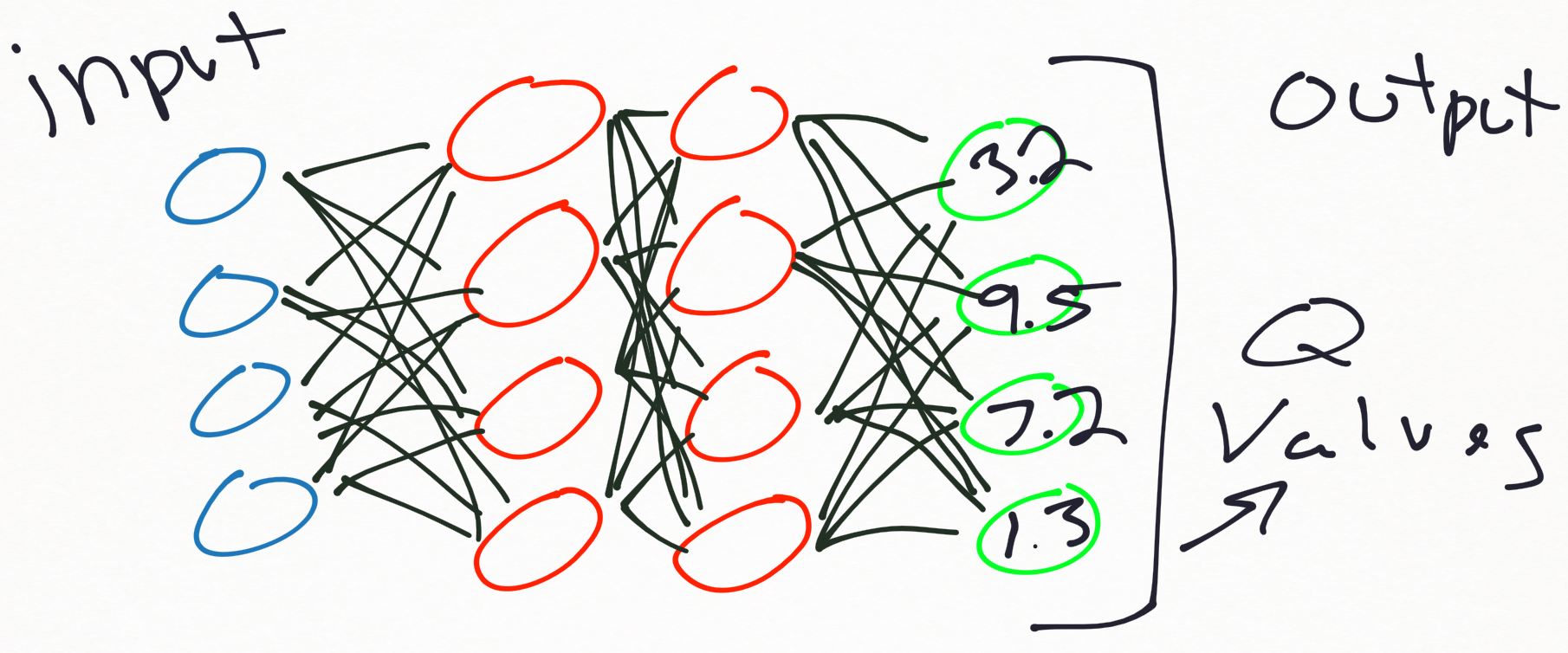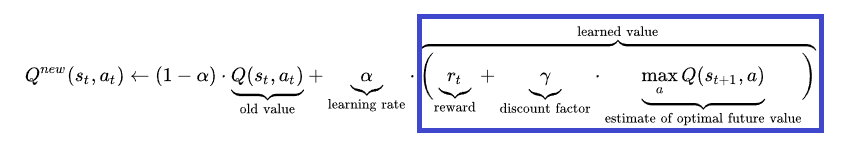## Deep Q Learning and Deep Q Networks (DQN) Intro and Agent - Reinforcement Learning w/ Python Tutorial p.5

Hello and welcome to the first video about Deep Q-Learning and Deep Q Networks, or DQNs. Deep Q Networks are the deep learning/neural network versions of Q-Learning.

With DQNs, instead of a Q Table to look up values, you have a model that you inference (make predictions from), and rather than updating the Q table, you fit (train) your model.

A typical DQN model might look something like:The DQN neural network model is a regression model, which typically will output values for each of our possible actions. These values will be continuous float values, and they are directly our Q values.

As we enage in the environment, we will do a `.predict()` to figure out our next move (or move randomly). When we do a `.predict()`, we will get the 3 float values, which are our Q values that map to actions. We will then do an argmax on these, like we would with our Q Table's values. We will then "update" our network by doing a `.fit()` based on updated Q values. When we do this, we will actually be fitting for all 3 Q values, even though we intend to just "update" one. There have been DQN models in the past that serve as a model per action, so you will have the same number of neural network models as you have actions, and each one is a regressor that outputs a Q value, but this approach isn't really used.

The formula for a new Q value changes slightly, as our neural network model itself takes over some parameters and some of the "logic" of choosing a value. Now, we just calculate the "learned value" part:With the introduction of neural networks, rather than a Q table, the complexity of our environment can go up significantly, without necessarily requiring more memory. As you can find quite quick with our Blob environment from previous tutorials, an environment of still fairly simple size, say, 50x50 will exhaust the memory of most people's computers. With a neural network, we don't quite have this problem. Also, we can do what most people have done with DQNs and make them convolutional neural networks. This effectively allows us to use just about any environment and size, with any visual sort of task, or at least one that can be represented visually. Up til now, we've really only been visualizing the environment for our benefit. Just because we can visualize an environment, it doesn't mean we'll be able to learn it, and some tasks may still require models far too large for our memory, but it gives us much more room, and allows us to learn much more complex tasks and environments. Once we get into DQNs, we will also find that we need to do a lot of tweaking and tuning to get things to actually work, just as you will have to do in order to get performance out of other classification and regression neural networks.

While neural networks will allow us to learn many orders of magnitude more environments, it's not all peaches and roses. Each step (frame in most cases) will require a model prediction and, likely, fitment (`model.fit()` and `model.predict()`. While calling this once isn't that big of a deal, calling it 200 times per episode, over the course of 25,000 episodes, adds up very fast. Thus, if something can be solved by a Q-Table and basic Q-Learning, you really ought to use that. This is true for many things. I have had many clients for my contracting and consulting work who want to use deep learning for tasks that really would actually be hindered by it. Keep it simple. For demonstration's sake, I will continue to use our blob environment for a basic DQN example, but where our Q-Learning algorithm could learn something in minutes, it will take our DQN hours. We will want to learn DQNs, however, because they will be able to solve things that Q-learning simply cannot...and it doesn't take long at all to exhaust Q-Learning's potentials.

DQNs first made waves with the Human-level control through deep reinforcement learning whitepaper, where it was shown that DQNs could be used to do things otherwise not possible though AI.

So let's start by building our DQN Agent code in Python.

```class DQNAgent:
def create_model(self):
model = Sequential()

model.add(Conv2D(256, (3, 3), input_shape=env.OBSERVATION_SPACE_VALUES))  # OBSERVATION_SPACE_VALUES = (10, 10, 3) a 10x10 RGB image.

model.add(Flatten())  # this converts our 3D feature maps to 1D feature vectors

model.add(Dense(env.ACTION_SPACE_SIZE, activation='linear'))  # ACTION_SPACE_SIZE = how many choices (9)
return model```

So far here, nothing special. It's your typical convnet, with a regression output, so the activation of the last layer is linear. If you do not know or understand convolutional neural networks, check out the convolutional neural networks tutorial with TensorFlow and Keras.

Now that that's out of the way, let's build out the `init` method for this agent class:

```    def __init__(self):

# Main model
self.model = self.create_model()

# Target network
self.target_model = self.create_model()
self.target_model.set_weights(self.model.get_weights())

# An array with last n steps for training
self.replay_memory = deque(maxlen=REPLAY_MEMORY_SIZE)

# Custom tensorboard object
self.tensorboard = ModifiedTensorBoard(log_dir="logs/{}-{}".format(MODEL_NAME, int(time.time())))

# Used to count when to update target network with main network's weights
self.target_update_counter = 0```

Here, you can see there are apparently two models: `self.model` and `self.target_model`. What's going on here? So every step we take, we want to update Q values, but we also are trying to predict from our model. Especially initially, our model is starting off as random, and it's being updated every single step, per every single episode. What ensues here are massive fluctuations that are super confusing to our model. This is why we almost always train neural networks with batches (that and the time-savings). One way this is solved is through a concept of memory replay, whereby we actually have two models.

The `target_model` is a model that we update every every `n` episodes (where we decide on `n`), and this the model that we use to determine what the `future Q values`.

Once we get into working with and training these models, I will further point out how we're using these two models. Eventually, we converge the two models so they are the same, but we want the model that we query for future Q values to be more stable than the model that we're actively fitting every single step.

Along these lines, we have a variable here called `replay_memory`. Replay memory is yet another way that we attempt to keep some sanity in a model that is getting trained every single step of an episode. We still have the issue of training/fitting a model on one sample of data. This is still a problem with neural networks. Thus, we're instead going to maintain a sort of "memory" for our agent. In our case, we'll remember 1000 previous actions, and then we will fit our model on a random selection of these previous 1000 actions. This helps to "smooth out" some of the crazy fluctuations that we'd otherwise be seeing. Like our `target_model`, we'll get a better idea of what's going on here when we actually get to the part of the code that deals with this I think.

The next thing you might be curious about here is `self.tensorboard`, which you can see is this `ModifiedTensorBoard` object. We're doing this to keep our log writing under control. Normally, Keras wants to write a logfile per `.fit()` which will give us a new ~200kb file per second. That's a lot of files and a lot of IO, where that IO can take longer even than the `.fit()`, so Daniel wrote a quick fix for that:

```from keras.callbacks import TensorBoard

#...

# Own Tensorboard class
class ModifiedTensorBoard(TensorBoard):

# Overriding init to set initial step and writer (we want one log file for all .fit() calls)
def __init__(self, **kwargs):
super().__init__(**kwargs)
self.step = 1
self.writer = tf.summary.FileWriter(self.log_dir)

# Overriding this method to stop creating default log writer
def set_model(self, model):
pass

# Overrided, saves logs with our step number
# (otherwise every .fit() will start writing from 0th step)
def on_epoch_end(self, epoch, logs=None):
self.update_stats(**logs)

# Overrided
# We train for one batch only, no need to save anything at epoch end
def on_batch_end(self, batch, logs=None):
pass

# Overrided, so won't close writer
def on_train_end(self, _):
pass

# Custom method for saving own metrics
# Creates writer, writes custom metrics and closes writer
def update_stats(self, **stats):
self._write_logs(stats, self.step)```

Finally, back in our DQN Agent class, we have the `self.target_update_counter`, which we use to decide when it's time to update our target model (recall we decided update this model every 'n' iterations, so that our predictions are reliable/stable).

Now for another new method for our `DQN Agent` class:

```    # Adds step's data to a memory replay array
# (observation space, action, reward, new observation space, done)
def update_replay_memory(self, transition):
self.replay_memory.append(transition)```

This just simply updates the replay memory, with the values commented above.

Next, we need a method to get Q values:

```    # Queries main network for Q values given current observation space (environment state)
def get_qs(self, state):
return self.model.predict(np.array(state).reshape(-1, *state.shape)/255)```

So this is just doing a `.predict()`. We do the reshape because TensorFlow wants that exact explicit way to shape. The -1 just means a variable amount of this data will/could be fed through.

Finally, we need to write our `train` method, which is what we'll be doing in the next tutorial!

The next tutorial:• Q-Learning introduction and Q Table - Reinforcement Learning w/ Python Tutorial p.1

• Q Algorithm and Agent (Q-Learning) - Reinforcement Learning w/ Python Tutorial p.2

• Q-Learning Analysis - Reinforcement Learning w/ Python Tutorial p.3

• Q-Learning In Our Own Custom Environment - Reinforcement Learning w/ Python Tutorial p.4

• Deep Q Learning and Deep Q Networks (DQN) Intro and Agent - Reinforcement Learning w/ Python Tutorial p.5
• Training Deep Q Learning and Deep Q Networks (DQN) Intro and Agent - Reinforcement Learning w/ Python Tutorial p.6